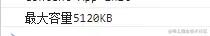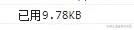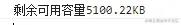﻿ JS代码计算LocalStorage容量示例详解 - 自由资讯

# JS代码计算LocalStorage容量示例详解

##### 目录
• LocalStorage 容量
• 计算总容量
• 已使用容量
• 剩余可用容量

## LocalStorage 容量

`localStorage`的容量大家都知道是`5M`，但是却很少人知道怎么去验证，而且某些场景需要计算`localStorage`的剩余容量时，就需要我们掌握计算容量的技能了~~

## 计算总容量

```let str = '0123456789'
let temp = ''
// 先做一个 10KB 的字符串
while (str.length !== 10240) {
str = str + '0123456789'
}
// 先清空
localStorage.clear()
const computedTotal = () => {
return new Promise((resolve) => {
// 不断往 LocalStorage 中累积存储 10KB
const timer = setInterval(() => {
try {
localStorage.setItem('temp', temp)
} catch {
// 报错说明超出最大存储
resolve(temp.length / 1024 - 10)
clearInterval(timer)
// 统计完记得清空
localStorage.clear()
}
temp += str
}, 0)
})
}
(async () => {
const total = await computedTotal()
console.log(`最大容量\${total}KB`)
})()
```## 已使用容量

```const computedUse = () => {
let cache = 0
for(let key in localStorage) {
if (localStorage.hasOwnProperty(key)) {
cache += localStorage.getItem(key).length
}
}
return (cache / 1024).toFixed(2)
}
(async () => {
const total = await computedTotal()
let o = '0123456789'
for(let i = 0 ; i < 1000; i++) {
o += '0123456789'
}
localStorage.setItem('o', o)
const useCache = computedUse()
console.log(`已用\${useCache}KB`)
})()
```## 剩余可用容量

```const computedsurplus = (total, use) => {
}
(async () => {
const total = await computedTotal()
let o = '0123456789'
for(let i = 0 ; i < 1000; i++) {
o += '0123456789'
}
localStorage.setItem('o', o)
const useCache = computedUse()
console.log(`剩余可用容量\${computedsurplus(total, useCache)}KB`)
})()
```﻿